# VCS Text Objects¶

Text objects allow you to insert text anywhere on a plot.

To download this Jupyter Notebook, right click on the link and choose "Download Linked File As..." or "Save Link as...". Remember where you saved the downloaded file, which should have an .ipynb extension. (You'll need to launch the Jupyter notebook or JupyterLab instance from the location where you store the notebook file.)

For this particular notebook, you will also need to download the sample font file, "FFF_Tusj.ttf", from the GitHub repository for this notebook: https://github.com/CDAT/Jupyter-notebooks/tree/master/vcs/VCS_Text_Objects. Save the font file in the same location as this notebook.

One additional file, "clt.nc" is needed for the notebook. Follow the instructions in the VCS Basics or VCS Principles notebooks to download the "clt.nc" sample data file. Alternatively, copy the following lines of code into a new cell early on in this notebook and run the cell which will download the files to a "sample data" directory within the conda environment you are using to run this notebook.

import vcs
import cdms2
import os
vcs.download_sample_data_files()

## Introduction¶

Back to Top

The first code block below does the following:

• imports the VCS library,
• creates and initializes an 800 px by 600 px canvas called canvas,
• creates a text object, txt, and
• lists all the attributes of the text object using txt.list().

As the output of the last line of code shows, a VCS text object is composed of two VCS objects: a Text Table object and a Text Orientation object.

In :
import vcs
canvas=vcs.init(geometry=(800,600),bg=True)
txt = vcs.createtext()
txt.list()

---------- Text combined (Tc) member (attribute) listings ----------
secondary method = Tc
---------- Text Table (Tt) member (attribute) listings ----------
Tt_name = __texttable_59297961364487
font = 1
spacing = 2
expansion = 100
color = [0.0, 0.0, 0.0, 100.0]
fillincolor = 0
priority = 1
string = ['']
viewport = [0.0, 1.0, 0.0, 1.0]
worldcoordinate = [0.0, 1.0, 0.0, 1.0]
x = None
y = None
projection = default
---------- Text Orientation (To) member (attribute) listings ----------
To_name = __textorientation_3585916609795
height = 14
angle = 0
path = right
halign = left
valign = half


The Text Table is mainly used to control the following:

• font: which font to use
• spacing: deprecated and not used
• expansion: deprecated and not used
• color: string or rgb[a]
• fillincolor: deprecated and not used
• priority: essentially the layer on the VCS canvas onto which to draw the text
• string: text to draw
• viewport: area on the canvas where VCS should draw the text object. Initialized at [0,1,0,1].
• worldcoordinate: corner values of the viewport area. Initialized at [0,1,0,1].
• x: x position (to be located using the worldcoordinate)
• y: y position (to be located using the worldcoordinate)
• projection: if you want to use a projection, this is the VCS projection object

The Text Orientation is mainly used to control the following:

• height: size of the text (font size)
• angle: rotation of the text (positive angle means rotating clockwise)
• path: deprecated, no longer used
• halign: horizontal alignment
• valign: vertical alignment

## Text Orientation¶

Back to Top

The following section shows how to control the different attributes of the Text Orientation object.

To get started, though, it is useful to understand the basics of the .x and .y positioning attributes from the Text Table, since they are used extensively throughout this notebook.

To plot the text string "Hello VCS User" in the center of the plot area, first assign the string to the .string attribute of the txt text object, then use the x and y attributes.

By default, the lower-left corner of the viewport (the area available for plotting) has an (x, y) coordinate of (0, 0) and (1, 1) for the upper-right corner. Therefore use .5 for the x and y coordinates to plot the text in the center.

In :
txt.string = 'Hello VCS User'
txt.x = .5
txt.y = .5
canvas.plot(txt)

Out: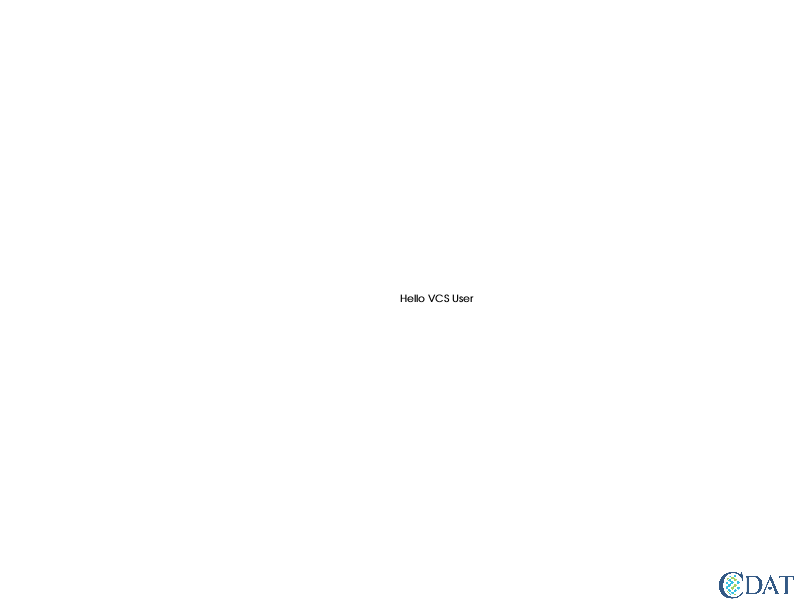### Size¶

Back to Top

You can control the size (or height) of text objects as follows:

In :
canvas.clear()
txt = vcs.createtext()
txt.string = "Example of BIG Text"
txt.x = .5
txt.y = .5
txt.height = 30
canvas.plot(txt)

Out: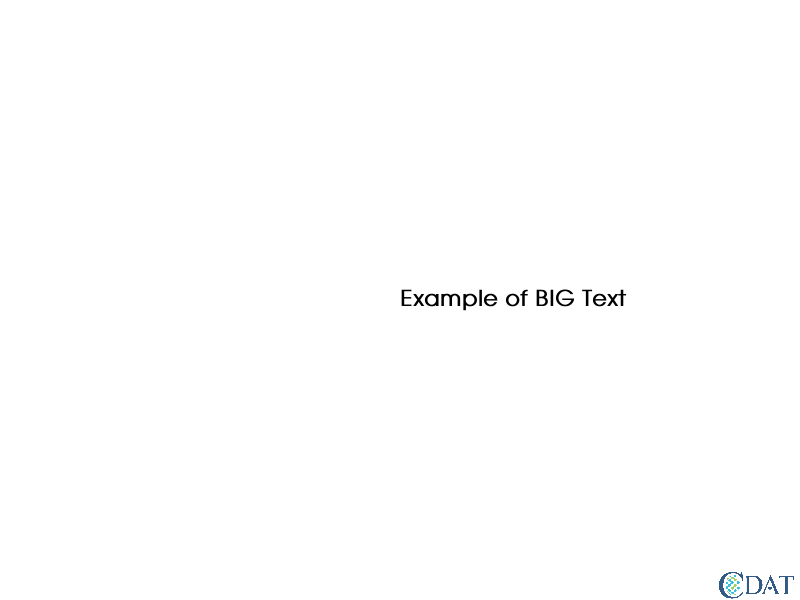### Angle¶

Back to Top

The .angle attribute controls the rotation of a text object from horizontal as shown below. A positive angle in degrees will rotate the text in a clockwise direction.

In :
canvas.clear()
txt.angle = 45
txt.height =20.
txt.string = "Rotated Text"
canvas.plot(txt)

Out: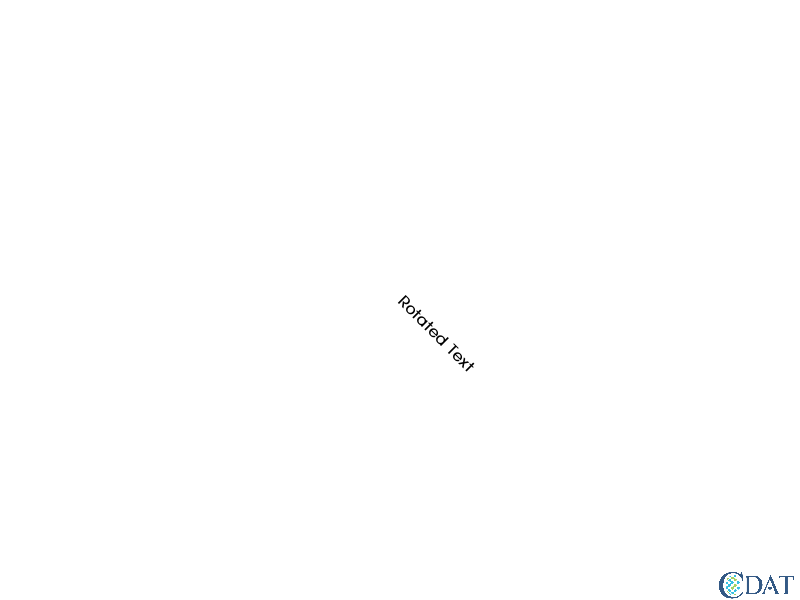### Alignment¶

Back to Top

You can control the alignment of text in both the horizontal and vertical directions.

Before exploring these options, let's reset the rotation back to horizontal with an angle value of 0.

In :
canvas.clear()
txt.angle = 0
txt.height =20.
txt.string = "Non-rotated Text"
canvas.plot(txt)

Out: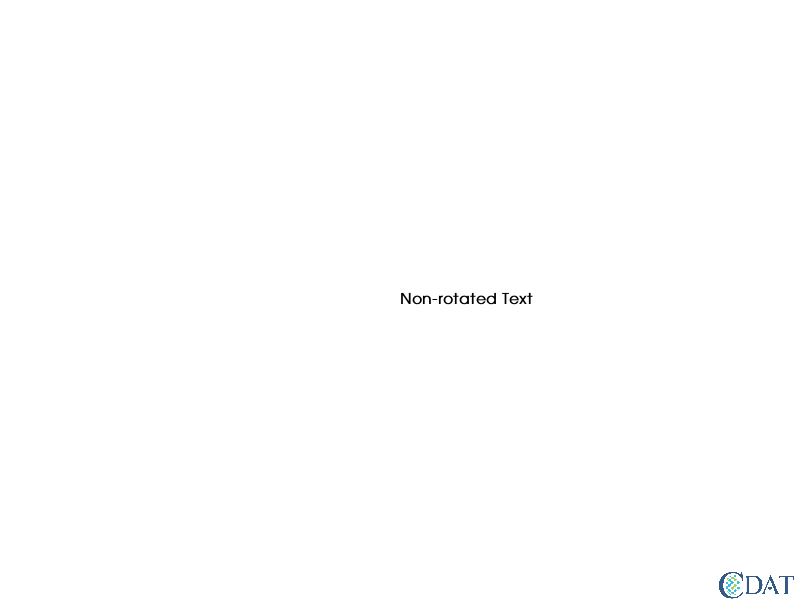#### Horizontal Alignment¶

Back to Top

You can control the horizontal alignment via the halign attribute. Possible values are: ('left', 'center', 'right') or (0, 1, 2).

In :
canvas.clear()
line = vcs.createline()
line.x = [.5,.5]
line.y = [0.,1.]
line.type ="dot"
line.color=["grey"]
center = vcs.createtext()
center.x = .5
center.y = .5
center.string = "Centered Text"
center.halign = "center"
right = vcs.createtext()
right.x = .5
right.y = .25
right.string = "Right Aligned Text"
right.halign = "right"
left = vcs.createtext()
left.x = .5
left.y = .75
left.string = "Left Aligned Text"
left.halign = "left"
canvas.plot(center)
canvas.plot(right)
canvas.plot(left)
canvas.plot(line)

Out: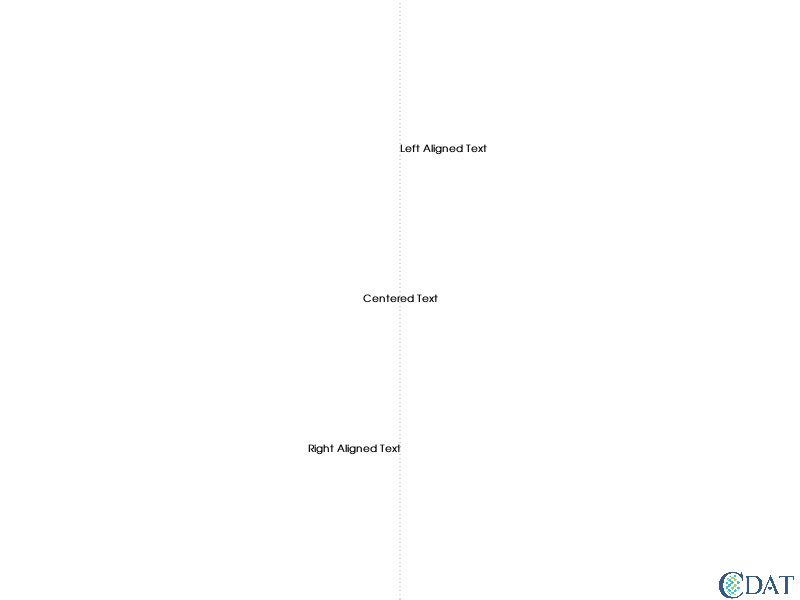#### Vertical Alignment¶

Back to Top

You can control the vertical alignment via the valign attribute. Possible values are: ('top', 'cap', 'half', 'base', 'bottom') or (0, 1, 2, 3, 4).

Note that currently, cap and top should not be used at the same time.

In :
canvas.clear()
line = vcs.createline()
line.y = [.5,.5]
line.x = [0.,1.]
line.type ="dot"
line.color=["grey"]

half = vcs.createtext()
half.height = 20
half.halign = "center"
half.x = .5
half.y = .5
half.string = "Half Aligned Text"
half.valign = "Half"

bottom = vcs.createtext()
bottom.halign='center'
bottom.height=20
bottom.x = .25
bottom.y = .5
bottom.string = "Bottom Aligned Text"
bottom.valign = "bottom"

top = vcs.createtext()
top.halign='center'
top.height=20
top.x = .75
top.y = .5
top.string = "Top Aligned Text"
top.valign = "top"

cap = vcs.createtext()
cap.x = .75
cap.y = .75
cap.string = "Cap Aligned Text"
cap.valign = "cap"

canvas.plot(half)
canvas.plot(bottom)
canvas.plot(top)
#canvas.plot(cap)
canvas.plot(line)

Out: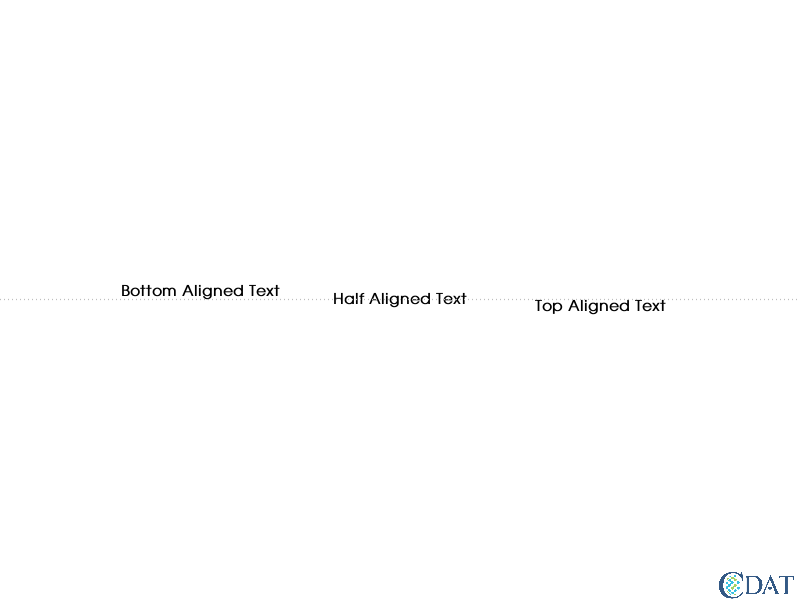## Text Table¶

Back to Top

The following section shows how to control the different attributes of the Text Table object.

### Fonts¶

Back to Top

#### Changing the Font¶

You can control the fonts via the font attribute.

For a list of available default fonts, use the vcs.listelements("font") option.

In :
print(vcs.listelements("font"))

['Adelon', 'Arabic', 'AvantGarde', 'Chinese', 'Clarendon', 'Courier', 'DejaVuSans', 'DejaVuSans-Bold', 'DejaVuSans-BoldOblique', 'DejaVuSans-ExtraLight', 'DejaVuSans-Oblique', 'DejaVuSansCondensed', 'DejaVuSansCondensed-Bold', 'DejaVuSansCondensed-BoldOblique', 'DejaVuSansCondensed-Oblique', 'Greek', 'Hebrew', 'Helvetica', 'Maths1', 'Maths2', 'Maths3', 'Maths4', 'Russian', 'Times', 'default']


In addition to names, fonts can be identified by their number. The first line of code below shows how to get the number of the "Times" font. The second line of code illustrates how to return the name of a font, given its number, "5" in this case.

In :
print("The 'Times' font number is:",vcs.getfontnumber("Times"))
print("The name of number 5 is:", vcs.getfont(5))

The 'Times' font number is: 6
The name of number 5 is: Adelon


### Default Font¶

Back to Top

If you wish to change VCS's default font, which will affect every object using font 1, you can use setdefaultfont.

In :
canvas.clear()
t = vcs.createtext()
t.string = "Default as default font"
t.x = .3
t.halign = "center"
t.y = .5
canvas.plot(t)
vcs.setdefaultfont("DejaVuSans-Bold")
t = vcs.createtext()
t.string = "DejaVuSans-Bold as default font"
t.x = .7
t.halign = "center"
t.y = .5
canvas.plot(t)

Out: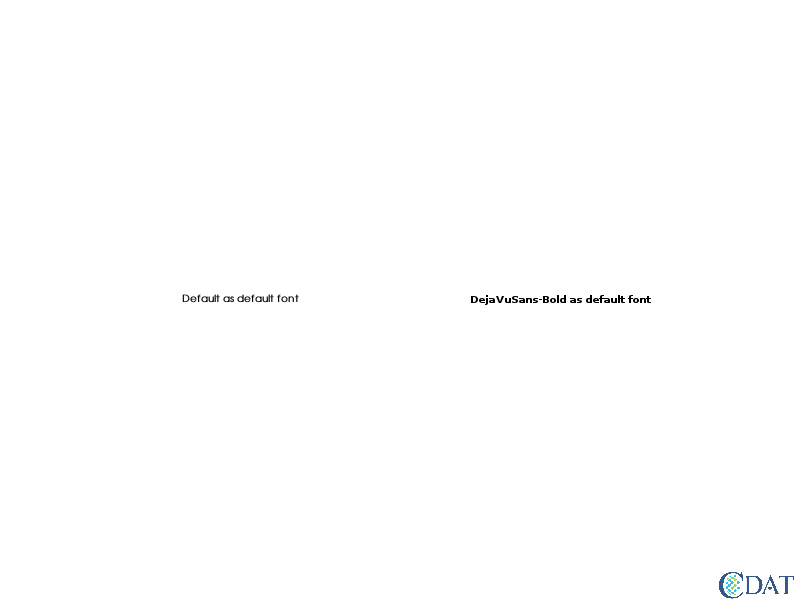Now let's reset the default font to AvantGarde from DejaVuSans-Bold and display all the different fonts in a grid. Since the font names will overlap each other when displayed, let's use an angle of 30.

In :
# reset default font to AvantGarde from DejaVuSans-Bold
vcs.setdefaultfont("AvantGarde")
txt.angle = 30
fonts = vcs.listelements("font")
N = len(fonts)
grid = 5. # 5x5 grid
delta = 1./6.
canvas.clear()
for i, font in enumerate(fonts):
print("I,f:",i,font)
txt.font = font
txt.string = font
yindx = i % grid
xindx = int(i/grid)
txt.x = delta + xindx*delta
txt.y = delta + yindx*delta
dsp = canvas.plot(txt)

dsp

I,f: 0 Adelon
I,f: 1 Arabic
I,f: 2 AvantGarde
I,f: 3 Chinese
I,f: 4 Clarendon
I,f: 5 Courier
I,f: 6 DejaVuSans
I,f: 7 DejaVuSans-Bold
I,f: 8 DejaVuSans-BoldOblique
I,f: 9 DejaVuSans-ExtraLight
I,f: 10 DejaVuSans-Oblique
I,f: 11 DejaVuSansCondensed
I,f: 12 DejaVuSansCondensed-Bold
I,f: 13 DejaVuSansCondensed-BoldOblique
I,f: 14 DejaVuSansCondensed-Oblique
I,f: 15 Greek
I,f: 16 Hebrew
I,f: 17 Helvetica
I,f: 18 Maths1
I,f: 19 Maths2
I,f: 20 Maths3
I,f: 21 Maths4
I,f: 22 Russian
I,f: 23 Times
I,f: 24 default

Out: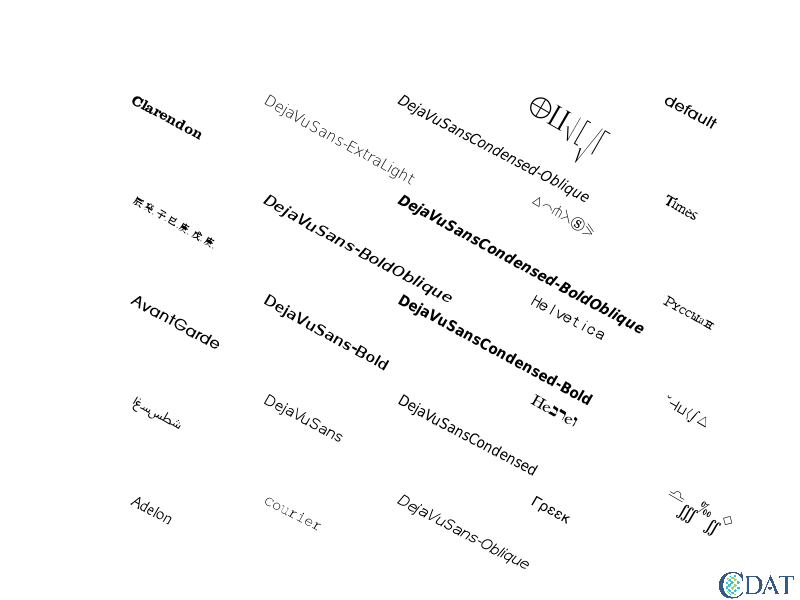To be ready for the next example, let's reset the canvas, text string, angle, font size (height), and plotting location.

In :
canvas.clear()
txt.string = "A VCS Text Object"
txt.angle = 0
txt.height = 12
txt.x = [.5]
txt.y = [.5]
canvas.plot(txt)

Out: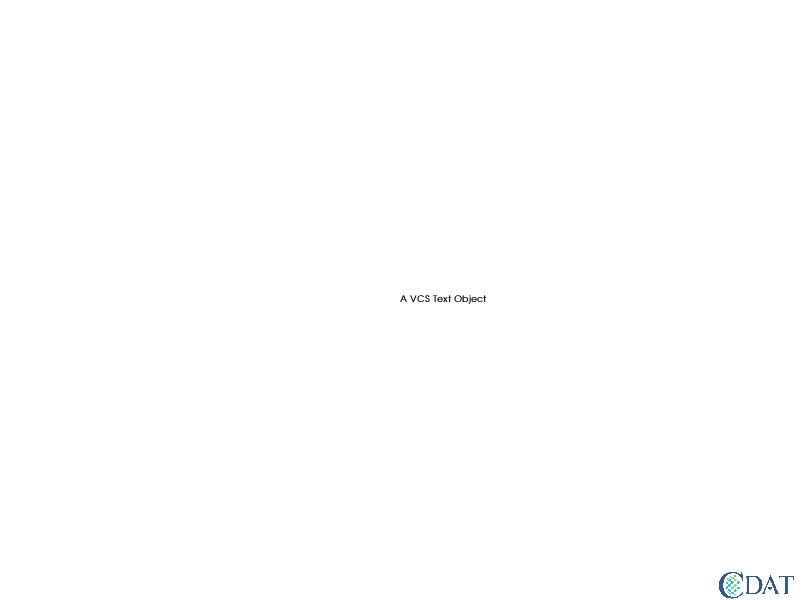#### Adding Fonts¶

Back to Top

You can add TrueType fonts to VCS by using the canvas.addfont function.

For the next lines of code to work properly, you need to download the sample font file, "FFF_Tusj.ttf", from the GitHub repo for this notebook: https://github.com/CDAT/Jupyter-notebooks/tree/master/vcs/VCS_Text_Objects. If you have not already done so, save the font file in the same location as this notebook.

In :
canvas.clear()
vcs.addfont("FFF_Tusj.ttf", name="Myfont")
txt.font = "Myfont"
canvas.plot(txt)

Out: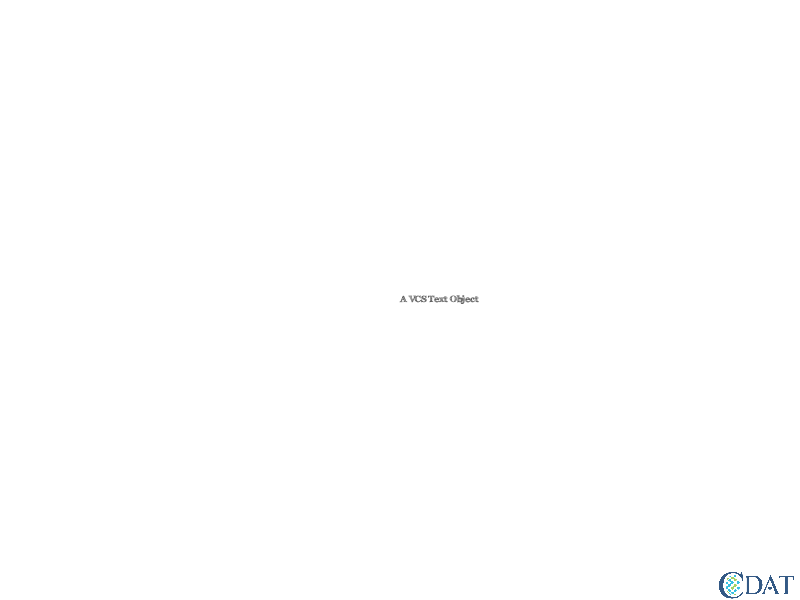### Color¶

Back to Top

You can control the font color via the color attribute. You can use a string representing the color name (e.g. "Red") or an index (e.g. 5) to set the color.

You can change the colormap via the colormap attribute.

Here we'll build a plot with the text string "A VCS Text Object" displayed in three different colors at three different horizontal (x) locations in the center of the plot.

First let's plot a red version in the center of the plot. Since the last time we assigned a value to txt.x it was 0.5 (txt.x = [.5]), we do not need to change that value here.

In :
canvas.clear()
txt.font = "default"
txt.color = "Red"
canvas.plot(txt)

Out: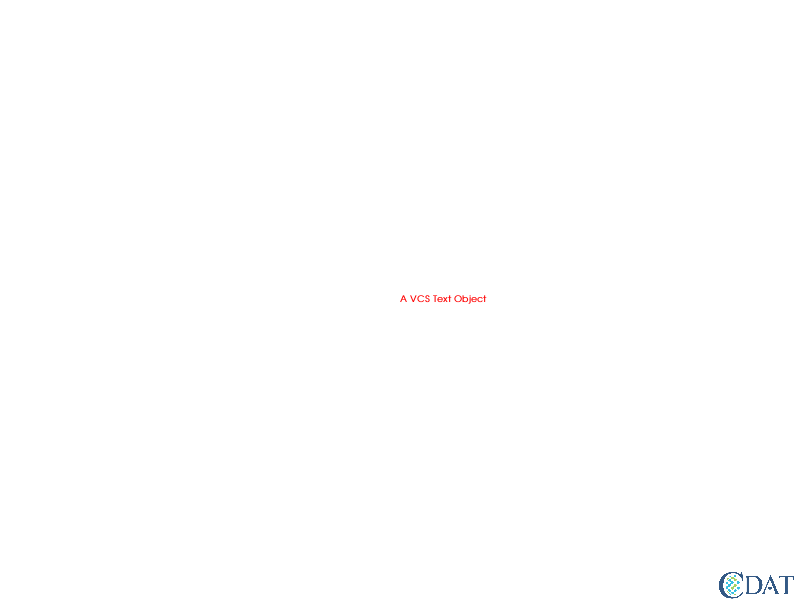Next we'll add the text string in color 5 from the default colormap (a purplish color), a bit to the right of the red string.

In :
txt.x += .2
txt.color = 5
canvas.plot(txt)

Out: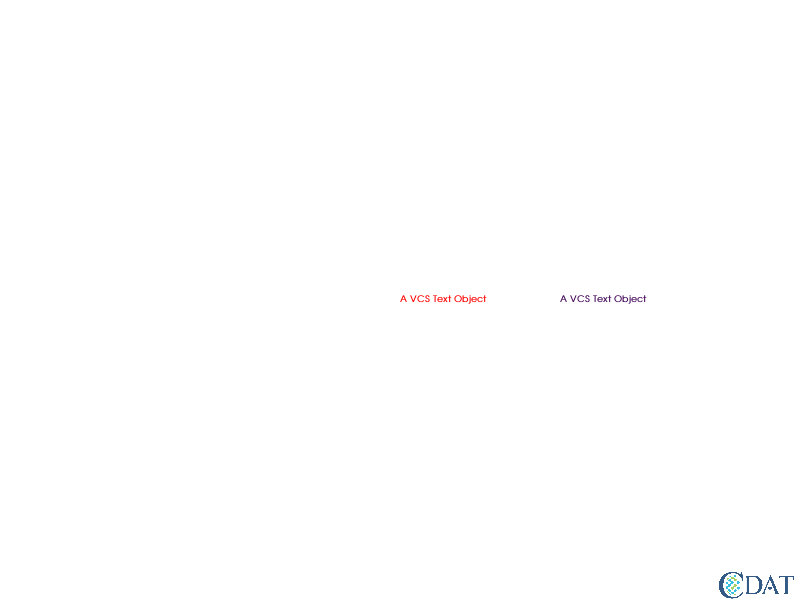Finally, we'll add a yellow version to the left of the red by changing the colormap from the default to "AMIP" which has a yellow color as index 5 as opposed to a purple color. The change in color is solely due to the change in the colormap.

In :
txt.colormap = "AMIP"  # Changing the colormap which will change the color of index 5
txt.x -= .4
canvas.plot(txt)

Out: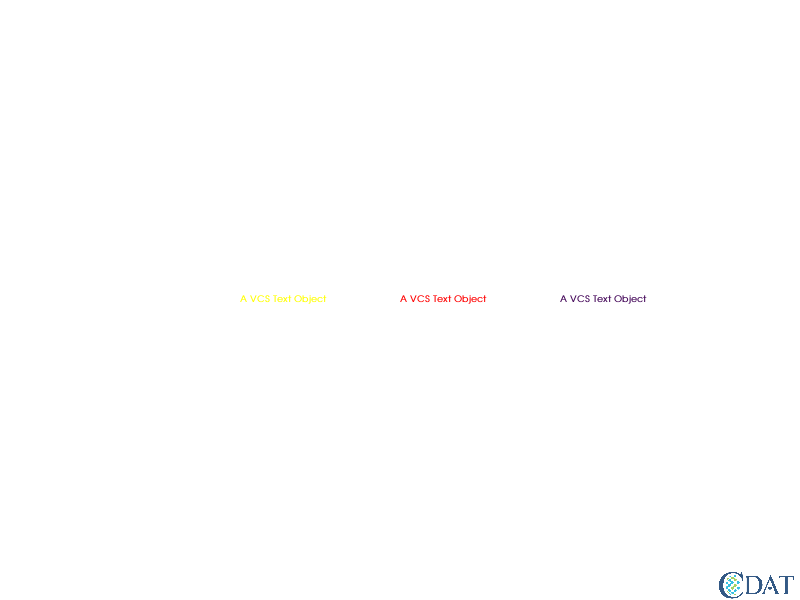### Viewport and World Coordinates¶

Back to Top

The world coordinate attribute determines the coordinate values at the corners of the plot area or viewport. By default the viewport covers the whole canvas.

As mentioned above, within the viewport, the default lower-left corner has an (x, y) coordinate of (0, 0) and the upper-right corner (1, 1).

#### Non-standard Viewport¶

Sometimes we do not want the coordinate system to cover the whole page, but rather an area where data is plotted.

As an example, to move the viewport to display in the lower, right section of the canvas use the following code:

In :
txt.viewport=[0.5,1,0,.5] # list the x bounds first, then y bounds
fa = vcs.createfillarea()
fa.x = [0.5, 1, 1, .5]
fa.y = [0, 0, .5, .5]
fa.color=[(80,80,80)]
fa.style="hatch"
fa.index=12
canvas.clear()
canvas.plot(fa)

txt.color="Red"
txt.height=15
canvas.plot(txt) # we do not need to tweak our text object coordinates at all

Out: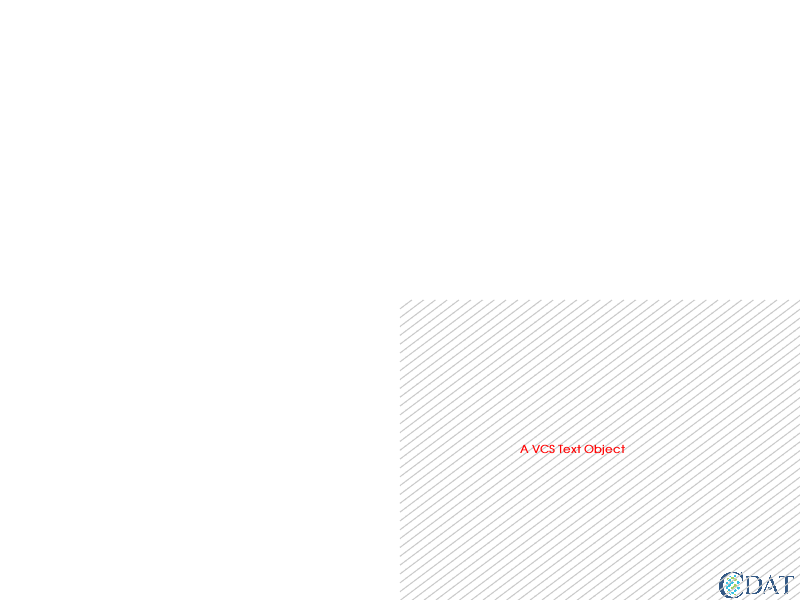#### Real-world Coordinates Example¶

Back to Top

Sometimes we would like to position the text based on actual, real-world coordinates rather than on a percentage of the page (e.g. .5 or 50%). In order to do this, we need to let VCS know about the world coordinates of its viewing area or viewport.

For context, let's plot a map of the Total Cloudiness, clt, over the contiguous United States from the "clt.nc" NetCDF file that is part of the VSC sample data set. Once we've plotted the data, we'll add a text object.

First we open the data file and read its contents into the f (for file) variable.

Next we define the latitude and longitude coordinates of our area of interest, the contiguous U.S.

We subset the total cloudiness data, create a template to use for plotting, and create an isofill of the data.

Next we define the bounds of the viewport and world coordinates.

Finally, we plot the subsetted data using the template. By specifying continents=3, the boundaries between Canada and Mexico are drawn as well as the U.S. state lines.

In :
canvas.clear()

import cdms2, os
f=cdms2.open(os.path.join(vcs.sample_data,"clt.nc"))

lon1 = -130.
lon2 = -70.
lat1= 25.
lat2 = 50
subset = f("clt",latitude=(lat1,lat2), longitude=(lon1, lon2))
tmpl = vcs.createtemplate()
iso = vcs.createisofill()
iso.datawc_x1 = lon1
iso.datawc_x2 = lon2
iso.datawc_y1 = lat1
iso.datawc_y2 = lat2

txt.viewport = [tmpl.data.x1, tmpl.data.x2, tmpl.data.y1, tmpl.data.y2]
txt.worldcoordinate = [lon1, lon2, lat1, lat2]

canvas.plot(subset,tmpl,iso, continents=3)

Out: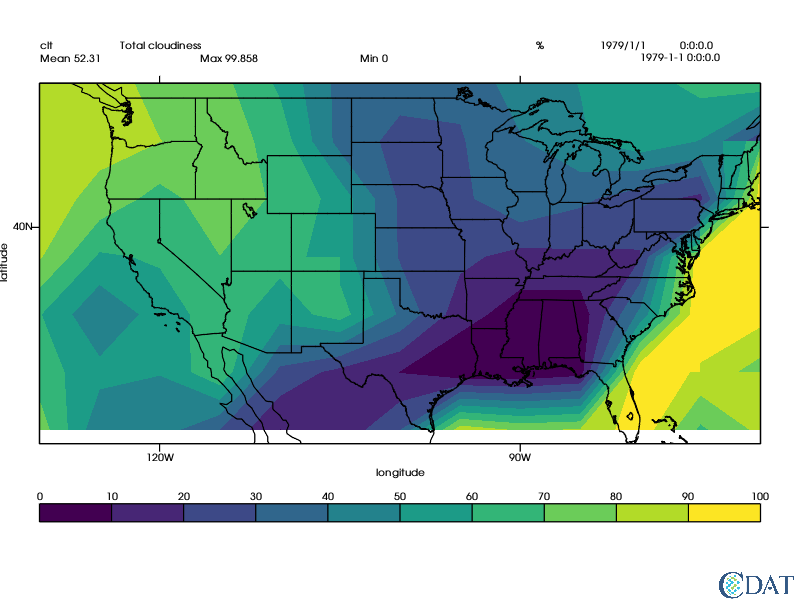Next we'll plot the location of Washington D.C. which is at -77.0369 latitude and 38.9072 longitude.

In :
txt.font = "default"
txt.color = "Red"
txt.height = 20
txt.halign = "center"
txt.valign = "half"
txt.x = -77.0369
txt.y = 38.9072
txt.string = "Washington D.C."
canvas.plot(txt)

Out: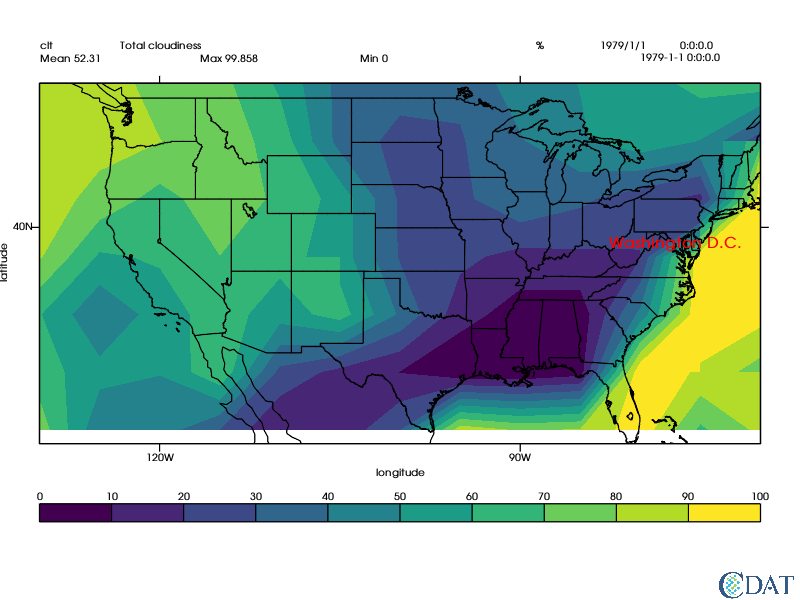### Projection¶

Back to Top

If your world coordinates represent latitude and longitude, you can use the projection attribute to apply a projection (and its settings) to your text location.

The following example again uses the Total Cloudiness sample data file, "clt.nc".

The final image includes two plots, the top of which plots the data on a rectangle covering the globe from -180 to 180 degrees longitude and -90 to 90 degrees latitude. The bottom, or second, plot uses a Polar projection.

The first use of the text object for plotting "Non proj" in blue on the top plot, does not use a projection, but does use the world coordinate. The second use of the text object to plot "PROJECTED" in red, does use the Polar projection.

In :
canvas.clear()

bot = canvas.gettemplate("bot_of2")
top = canvas.gettemplate("top_of2")
gm = canvas.createisofill()
gm.datawc_x1 = -180
gm.datawc_x2 = 180
gm.datawc_y1 = -90
gm.datawc_y2 = 90
canvas.plot(f("clt", slice(0,1)),gm,top)

proj = "polar"
gm.projection = proj
canvas.plot(f("clt",slice(0,1),longitude=(-180,181)),gm,bot)

txt = vcs.createtext()
txt.string = "Non proj"
txt.worldcoordinate = [-180,180,-90,90]
txt.x = -30
txt.y = 80
txt.color="blue"
txt.height = 15
txt.halign = "center"
txt.viewport = top.data.x1, top.data.x2, top.data.y1, top.data.y2
canvas.plot(txt)

txt.projection = proj
txt.color = "red"
txt.string = "PROJECTED"
txt.viewport = bot.data.x1, bot.data.x2, bot.data.y1, bot.data.y2
canvas.plot(txt)

Out: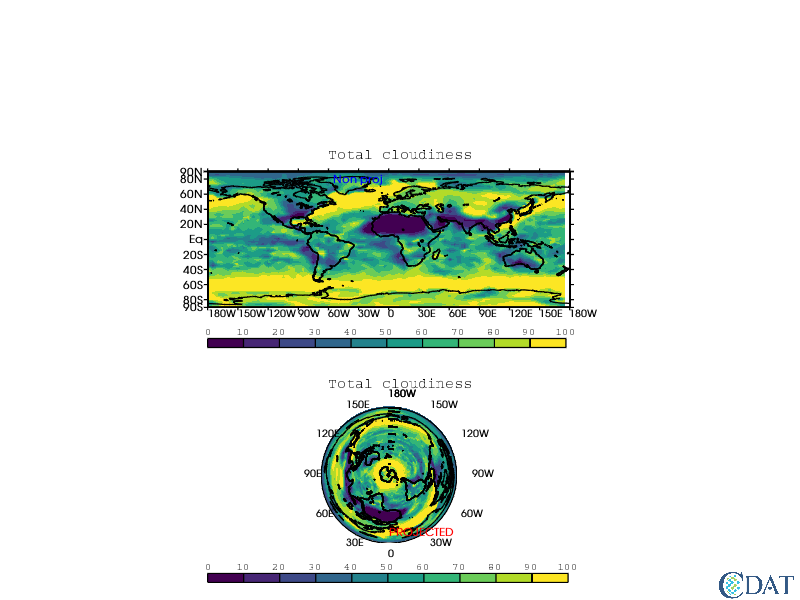### Ordering Plot Layers¶

Back to Top

You can control the layer on which the object will be drawn via the priority attribute. Objects with a priority value that is numerically larger than other objects (e.g. 2 instead of 1) are drawn on top of objects with smaller priority values.

In :
txt.priority = 0  # Turn off
txt.priority = 2  # Move to a layer on top of the "default" layer (1)


### Deprecated Attributes¶

The following attributes are left over from XGKS and are not used anymore:

spacing, fillingcolor, expansion

## Mathematical Expressions and Symbols¶

Please see the separate notebook dedicated to plotting Mathematical Expressions and Symbols.

## Example: Bringing It All Together¶

Back to Top

Let's plot the Total Cloudiness over the contiguous United States using real-world coordinates and a Lambert projection, then plot a colored text object containing three city names on top of the map.

In :
canvas.clear()

# Continental U.S.A. region
lat1 = 15.
lat2 = 70.
lon1 = -140.
lon2 = -60.
proj = "lambert"

# Read this subset of data in
clt = f("clt",time=slice(0,1),latitude=(lat1,lat2),longitude=(lon1,lon2),squeeze=1)

# Use the Isofill method to plot the total cloudiness data
gm = vcs.createisofill()
gm.datawc_x1 = lon1
gm.datawc_x2 = lon2
gm.datawc_y1 = lat1
gm.datawc_y2 = lat2
gm.projection = proj

# Use the default template, but don't modify it
templ = vcs.createtemplate()

# Create and define the text object
txt = vcs.createtext()
txt.string = ["Washington D.C.", "New York", "Los Angeles"]
txt.halign = "center"
txt.valign = "half"
txt.color = "red"
txt.font = "Myfont"
txt.height = 15
txt.priority = 2
txt.angle = -5
txt.y = [38.9072, 40.7128, 34.0522]
txt.x = [-77.0369, -74.0060, -118.2437]
txt.worldcoordinate = [lon1, lon2, lat1, lat2]
txt.viewport = [templ.data.x1, templ.data.x2, templ.data.y1, templ.data.y2]
txt.projection = proj

canvas.plot(clt,gm)
canvas.plot(txt)

Out: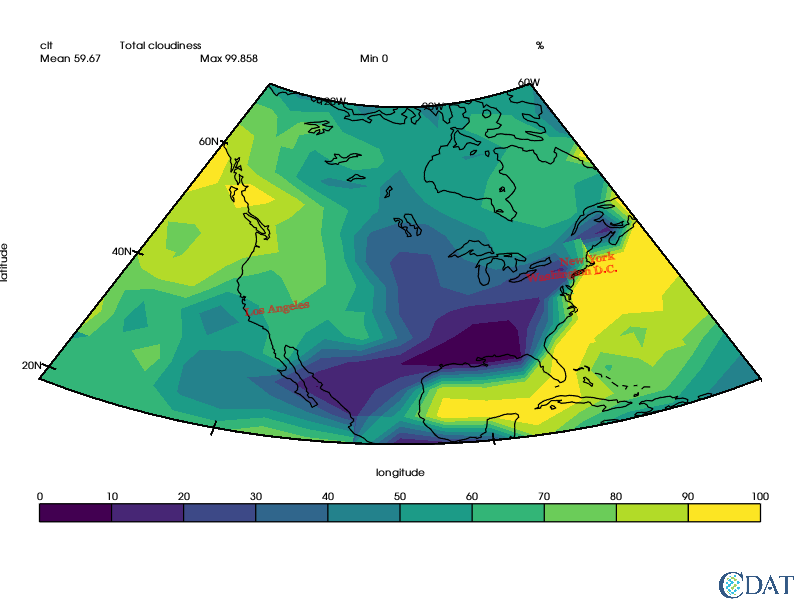The CDAT software was developed by LLNL. This work was performed under the auspices of the U.S. Department of Energy by Lawrence Livermore National Laboratory under Contract DE-AC52-07NA27344.

If you have questions about this notebook, please email our CDAT Support address, cdat-support@llnl.gov.